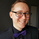# P-Square - Estimation of the Nth percentile of a series

1106 vizualizações
Estimation of the Nth percentile of a series
When working with built-in functions in TradingView we have to limit our length parameters to max 4999. In case we want to use a function on the whole available series (bar 0 all the way to the current bar), we can usually not do this without manually creating these calculations in our code. For things like mean or standard deviation, this is quite trivial, but for things like percentiles, this is usually very costly. In more complex scripts, this becomes impossible because of resource restrictions from the Pine Script execution servers.

One solution to this is to use an estimation algorithm to get close to the true percentile value. Therefore, I have ported this implementation of the P-Square algorithm to Pine Script. P-Square is a fast algorithm that does a good job at estimating percentiles in data streams. Here's the algorithms original paper.

The chart
On the chart we see:
• The returns of the series (blue scatter plot)
• The mean of the returns of the series (orange line)
• The standard deviation of the returns of the series (yellow line)
• The actual 84.1th percentile of the returns (white line)
• The estimatedl 84.1th percentile of the returns using the P-Square algorithm (green line)

Note: We can see that the returns are not normally distributed as we can see that one standard deviation is higher than the 84.1th percentile. One standard deviation should equal the 84.1th percentile if the data is normally distributed.
Script de código aberto

No verdadeiro espírito TradingView, o autor deste script o publicou com código aberto, para que os traders possam compreendê-lo e verificá-lo. Um brinde ao autor! Você pode usá-lo gratuitamente, mas a reutilização deste código em uma publicação é regida pelas Regras da Casa. Você pode favoritá-lo para utilizá-lo em um gráfico.

Quer usar esse script no gráfico?

## Comentáriosgmeneguzzo
@gmeneguzzo, I guess you could use this as is for measuring risk. For instance, use this on the daily time frame with the percentile setting set to 90 and you'll know approximately how far price moves 90% of the days that TradingView has data for. This can help you with risk management and money management when you day trade the opening of a session for example.
Resposta